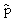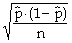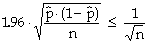## Estimating a Proportion

In order to estimate the fraction (percentage, proportion) of individuals in the population who possess a certain characteristic, let those individuals with the characteristic be labeled as ‘1’s, and those without the characteristic as ‘0’s, and estimate the population mean of this artificial (“dummy”) variable. (The mean is the number of ‘1’s, divided by the population size – This is the proportion sought.)

 the sample proportion (mean)the sample standard deviationthe standard error of the proportionthe margin of error of the estimateFor example, assume that 400 recent purchasers of a product are contacted, and 240 are female. Estimate the fraction of all recent purchasers who are female.

Answer:= 0.6 = 60%, with a margin of error of 0.048 = 4.8%

### A Bit More on Estimating Proportions

It's worth noting that, when a proportion is being estimated, the margin of error (at the 95%-confidence level) is always somewhat less than 1/√n. This can be useful when interpreting survey data.

This article provides some cautions concerning the interpretation (in the popular press) of political polls.